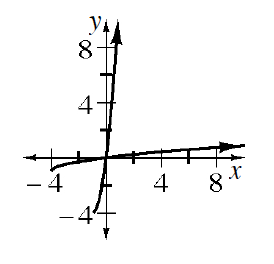### Home > INT3 > Chapter 9 > Lesson 9.1.4 > Problem9-58

9-58.

For the function $f(x) = \frac { \sqrt { x + 4 } } { 2 }-1$, complete parts (a) through (c) below.

1. Sketch the graph and its inverse.

$\text{Recall the parent function }f(x)=\sqrt{x}.$2. What is the equation of the inverse function?

Have $x$ and $y$ switch places in the equation and solve for $y$.

3. Determine the domain and range of the inverse.

Notice from your answer in part (a) the direction where the lines continue.